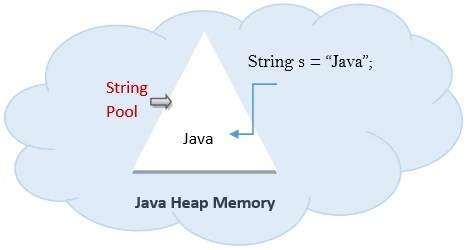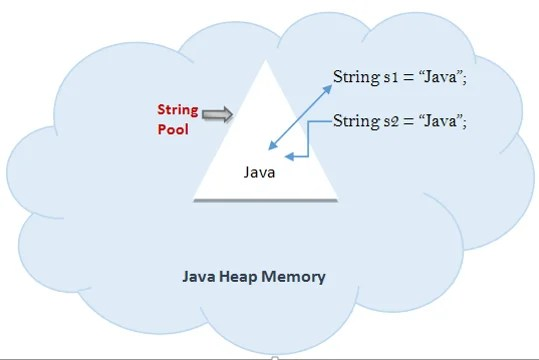## Learn String operations in Java using examples

In the previous article, we discussed the String class in Java. We learnt the ways a String object can be constructed. We also figured out the difference between String and StringBuffer. We discussed 2 string methods length() and Intern() as well. I hope that the article would have been helped you in the endeavours learning Strings in Java.

If you are preparing for any automation related interview then you should read Commonly asked java programs in Selenium Interview
and you should read this post which covers the most common interview question related to String

5 Commonly Asked Java String Question in Selenium Interview

In continuation here we will talk about some more string operation (mostly useful for automation testing practice) through which String can be manipulated in the many ways according to the need. In automation testing, we face many situations where it is required to manipulate the string.

1. Concatenating String: In Java, two or more string objects can be concatenated in 2 ways as below:
1. Using + operator: Please take a look in the below program:

//another example

2. Using concat() method: Please refer to below example:

2. CharAt: This method helps us to find the character at the given index of the String. The index starts from 0 i.e. the first character of the string is at the 0th index. The last character is at the length() – 1 index. (Syntax: public char charAt(int index) )

3. compareTo: This method is used to compare the two strings lexicographically. It returns int value as the comparison result.
Value ‘0’ is returned if both the strings are equal. Value less than ‘0’ is returned if the argument string is greater than this string. A value greater than ‘0’ is returned if the argument string is less than this string.

CompareTo method can also be used to compare any 2 objects as this method is part of Java object class. So it is not limited to String comparisons only.
4. ContentEquals: This method compares the String with StringBuffer and returns a Boolean value.

5. Equals and equalsIgnoreCase: To compare two strings for equality, use equals( ). It has this general form:

Here, str is the String object is being compared with the invoked String object. It returns true if the strings contain the same characters in the same order, and false otherwise. The comparison is case-sensitive. To perform a comparison that ignores case differences, call equalsIgnoreCase().

When it compares two strings, it considers A-Z to be the same as a-z. It has this general form:

Here, str is the String object being compared with the invoking String object. It, too, returns true if the strings contain the same characters in the same order, and false otherwise.
Here is an example that demonstrates equals( ) and equalsIgnoreCase( ):

The output from the program is shown here:

6. getChars: If you need to extract more than one character at a time, you can use the getChars( ) method. It has this general form:

Here is the output of this program:

7. replace: The replace( ) method replaces all occurrences of one character in the invoking string with another character. It has the following general form:

Here, original specifies the character to be replaced by the character specified by replacement. The resulting string is returned. For example,

puts the string “Hewwo” into s.

8. split:  Ahhh!! I just love this method. I have built a framework around this function only.
You can split a String using this method. The string is broken around the given delimiter(regular expression). It returns an array of Strings. Let’s understand by an example:

Here is the output of this program:

Number of splited Strings can also be controlled by using the overloaded method of Split

output:

9. substring: You can extract a substring using substring( ). It has two forms. The first is

Here, startIndex specifies the index at which the substring will begin. This form returns a copy of the substring that begins at startIndex and runs to the end of the invoking string. The second form of substring( ) allows you to specify both the beginning and ending index of the substring:

Here, startIndex specifies the beginning index, and endIndex specifies the stopping point. The string returned contains all the characters from the beginning index, up to, but not including, the ending index.

output:

10.  toUppercase and toLowerCase: These 2 methods are used to convert the questioned string to all upper case letters and lower case letters respectively.

11. Trim: If there is a situation where you have the string which has spaces in its starting and in ending, this method will help you out to remove those.

If you want to explore more about String then read it from here

## Exploring String class in Java

In Java, String class is a sub-class of java.lang and there are available to all programs automatically. Java implements String as objects of String unlike other programming language which implements it as character arrays.

### String Constructors:

1. The string class can be initialized using several constructors. To create an empty string, default constructor is as follows:

Above will create an instance of String with no characters in it.

1. To create a String containing character sequence use below constructor:

`String(char chars[ ])`

For example:

`char chars[] = { 'a', 'b', 'c' };`

String s = new String(chars);

In the above example a string object will be created having ‘abc’ as its value.

1. String object can also be passed as constructor argument.

`String(String strObj)`

See below example:

`class MakeString {`

`public static void main(String args[]) {`

`char c[] = {'J', 'a', 'v', 'a'};`

`String s1 = new String(c);`

`String s2 = new String(s1);`

`System.out.println(s1);`

`System.out.println(s2);`

`}`

`}`

The output from this program is as follows:

`Java`

`Java`

As you can see, s1 and s2 contain the same string.

1. String class provides constructors that initialize a string when given a byte array.

`String(byte asciiChars[ ])`

`class SubStringCons {`

`public static void main(String args[]) {`

`byte ascii[] = {65, 66, 67, 68, 69, 70 };`

`String s1 = new String(ascii);`

`System.out.println(s1);`

`}`

`}`

This program generates the following output:

`ABCDEF`

1. String can also be initialized using below code as well:

`String s = new String(“JAVA”);`

### String Length:

The string length is the number of characters in contains. This value can be obtained using length() method. Its return type is int.

`String s = new String(“JAVA”);`

`System.out.println(s.length());`

The above will print 4 because there are 4 characters in the string “JAVA”.

### String Literals:

We saw that how a string can be constructed through array of characters using new keyword. Java provides simpler way to construct a string using literals. For each string literal in the program Java automatically constructs a string object.

`String s = “Java”;`

The above will create a string object and it can be used all the string operations and manipulations in the program. Like below line of code will print string length as 4.

`System.out.println(s.length());`

Difference between initializing a string by literal and by new keyword:

Before going into the details let’s understand the Java Heap and String Pool. Those are basically memory spaces that JVM keeps with itself since its startup. Before Java 1.7 these are two separate memory allocations but from Java 1.7 onwards String pool occupies some space from Java Heap itself. Please see the below diagram.String Pool is a memory pool inside Java heap where String value created by literal will be placed out.

`String s = "Java";`

When above code will be executed then it will look into the String pool for the same value. If it is found then s will refer to that but if it is not found then a new String with value (Java as in case) will be created and s will refer to this value. So if we will create another string in the pool with the same value like below:

`String s1 = "Java";`

It will not create any new string in the pool but will refer to the string created by previous code.

Consider below lines of code:

`String s = "abodeqa";`

`String s1 = "abodeqa";`

`System.out.println( s==s1 );`

Above will print true because both the string objects are referring to the same memory location. But when string object is created using new keyword:

`String s1 = new String ("Java");`

then string object will be created in the Java Heap but not in string pool.also if another string object is created using new keyword having same value it will create another fresh object in Java heap unlike the literal. See above diagram.

`String s1 = new String("abodeqa");`

`String s2 = new String("abodeqa");`

`String s3 = "abodeqa";`

`System.out.println( s1==s2 ); // return false because both the object are referring to different memory locations`

`System.out.println( s1==s3 ); // return false because s1 is in heap and s3 is in string pool`

`System.out.println( s1.equalsTo ); // return true`

We can use intern() method on the string created by new keyword. This will create the string in the string pool.

`String s = "Java";`

`String s1 = new String("Java");`

`String s2 = new String("Java").intern();`

`System.out.println(s==s2); // true`

`System.out.println(s==s1); //false`

`System.out.println(s1==s2); //false`I hope above explanation is understandable. If you have doubts please comment on the same. It will be answered to the best of my knowledge.